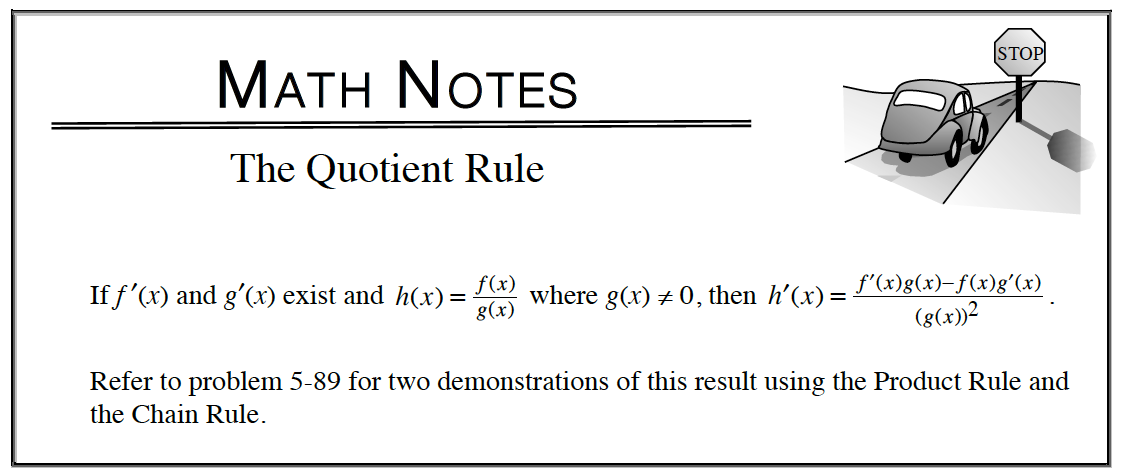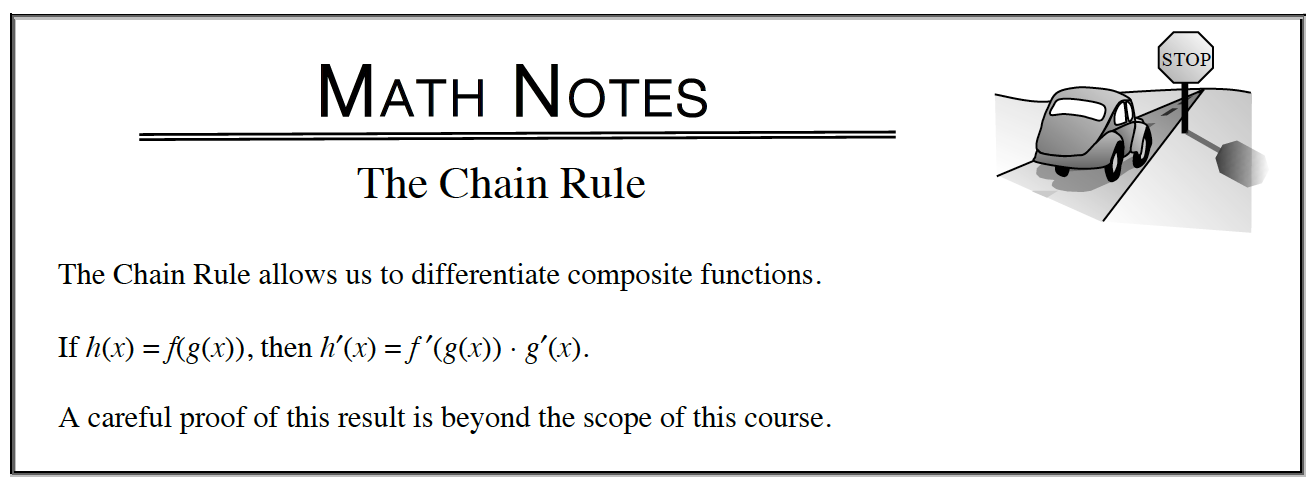Home > APCALC > Chapter 7 > Lesson 7.4.2 > Problem7-194

7-194.

Differentiate each of the following equations. Homework Help ✎

1.  $y =\operatorname { sin } ^ { - 1 } ( \sqrt { 1 - x } )$

Be mindful about positive and negative values.

1. $y =\frac { 2 \operatorname { cos } ( x ) } { \sqrt { 1 + x ^ { 2 } } }$

Quotient Rule1. $y = xe^{sec(x)}$

Chain Rule$\text{Recall that }\frac{d}{dx}\sec(x)=\sec(x)\tan(x).$

1. $\ln(y) = x \ln(x)$

Use implicit differentiation.